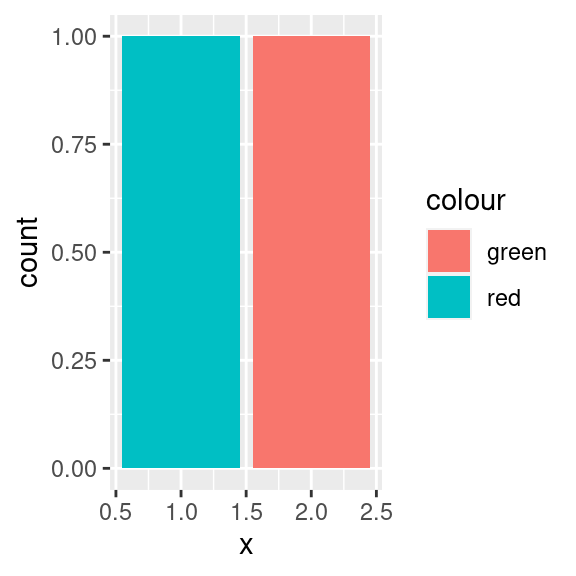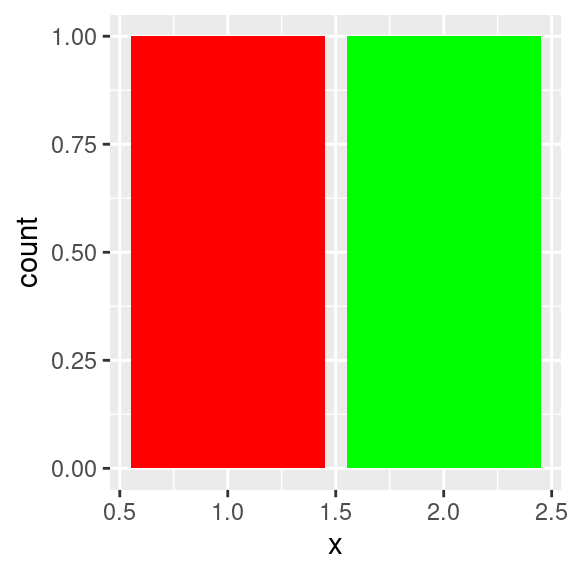# The I()dentity strategy

## What’s the pattern?

One simple, but convenient, strategy is to use the base `I()` function to create objects of class `AsIs`. These are useful for representing values that should remain as is, when the default might be to change them in some way.

## What are some examples?

There are two places that you can use `I()` in base R:

• When creating a data frame, you can use it to request that `data.frame()` not transform the column in any way. It’s one way you can create a list-column in base R:

``````x <- list(1, 2:3, 4:6)

# By default, if you give a data frame a list it will try to make
# each element a column:
data.frame(x = x)
#> Error in (function (..., row.names = NULL, check.rows = FALSE, check.names = TRUE, : arguments imply differing number of rows: 1, 2, 3

# But if you wrap it in `I()` it will become a list-column
data.frame(x = I(x))
#>         x
#> 1       1
#> 2    2, 3
#> 3 4, 5, 6``````
• When fitting a linear model, `I()` allows you to escape the usual Wilkinson-Rogers interpretation of addition and multiplication and instead created a transformed input:

``````# fit a model with three terms: x, y, x:y
lm(z ~ x * y)

# fit a model with one term: x * y
lm(z ~ I(x * y))``````

You’ll see `I()` used in a variety of places in the tidyverse:

• In readr, you can use `I()` to indiate that you are supplying a string containing the literal data, rather than a path giving where to find the data:

``````readr::read_csv(I("x,y\n1,2"))
#> # A tibble: 1 × 2
#>       x     y
#>   <dbl> <dbl>
#> 1     1     2``````
• In ggplot2, you can use it to indicate that the values don’t need to be transformed; they’re the literal aeshetic values already. For example, compare the following two plots:

``````library(ggplot2)

df <- data.frame(x = 1:2, colour = c("red", "green"))

df |> ggplot(aes(x, fill = colour)) + geom_bar()
df |> ggplot(aes(x, fill = I(colour))) + geom_bar()``````• httr2, a tool for generating HTTP requests, will automatically escape special characters when constructing a URL. You can use `I()` to say that you’ve already escaped the string and it doesn’t need further escaping.

### How can I use it?

`I()` wraps adds the `"AsIs"` class to the object it wraps, so you can detect if `I()` has been used by checking for `inherits(x, "AsIs")`.

It’s best used for simple cases where there are two possible interpretations for an argument and one of them has more of a sense for being untransformed or unaltered in some way. For example, you could imagine using it instead of `fixed()` in stringr if there was only a choice between regular expressions and fixed stringr, but it’s not quite powerful.

If you’re using `I()` for escaping, it’s good practice to wrap any escaped values in `I()` to indicate that you’ve escaped them. That ensures that you never accidentally double-escape an input. For example, this is how you might write code like what httr2 uses to escape query parameters.

``````escape_params <- function(x) {
if (inherits(x, "AsIs")) {
x
} else {
I(curl::curl_escape(x))
}
}

x <- escape_params("Good morning")
x
#>  "Good%20morning"``````

Wrapping the output in `I()` ensures that no matter how many times we call `escape_params()` the string is only escaped once. This is a particularly useful property as your code starts to get more complicated.

``````escape_params(x)
#>  "Good%20morning"``````

You can see here one of the downsides of using `I()`: the printed output of wrapped objects are no different from the objects themselves leaving to potentially confusing behaviour when two seemingly identical inputs yield different outputs.